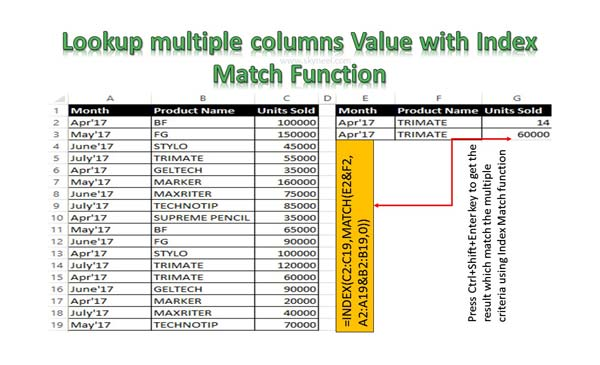# Lookup multiple columns Value with Index Match Function

If you have multiple criteria then you can Lookup multiple columns value using Index Match Function at place of VLookup function in Excel.

To lookup multiple column values you can use INDEX MATCH function in Excel. During using the multiple criteria, you can use an array formula to get the accurate result. In this guide we have to show you strength of Excel Index Match function. Sometime VLookup function does not provide correct result in that situation you have to use Index Match function.

In this guide we show you how Index Match function is better than VLookup function in Excel. There are lot of built-in function in Excel and each function has it’s own importance. If any function does not able to get the correct result as per your need then you have to use more than one functions at once. VLookup function is best function to lookup the values in same or other worksheet. But Index Match function is an alternative way which helps you to get the result when VLookup fails. This guide allows you to quickly use Index Match function with multiple criteria in active Excel sheet.

### Index Function in Excel

Index function return a value or the reference number to a value from given range of cells or table array.

INDEX(array, row_num, [column_num])

Array : It is a range of cells or an array constant.

Row_num : Selects the row number in an array from which to return a value. If Row_num is omitted, Column_num is required.

Column_num : It is an optional. Selects the column number in an array from which to return a value. If Column_num is omitted, Row_num is required.

### Match Function in Excel

This function is used to returns the relative position of an item in the given array or range of cells that match a specific value in specific order.

MATCH (lookup value, lookup array, [match_type] )

lookup_value: The value that you want to match in lookup_array. The lookup_value argument can be any number, text, logical value or a cell reference to a number, text, or logical value.

lookup_array: It is range of cells being searched.

match_type: It is an optional. The number -1, 0, or 1. The match_type argument specifies how Excel matches lookup_value with values in lookup_array. By default value for this argument is 1.

For eg: You have a large worksheet data in which you have Month name, Product name and number of units sold. Now you want to lookup value number of units sold which match month and product name.

## Step to Lookup multiple columns Value with Index Match Function

Index Match is an advanced function which allows you to use multiple criteria to quickly get the result. To do this job you have to take given following steps.Step 1: Prepare the following given structure to know how to lookup multiple columns value with Index Match function.

Step 2: To lookup number of units sold which match the given month name and product name in cell E3 and F3 respectively. To do this job you have to type given function in cell G2 =INDEX(C2:C19,MATCH(E2&F2,A2:A19&B2:B19,0)). Now if you press enter key then you will get #VALUE! error value as a result.

So press Ctrl+Shift+Enter shortcut key to get the accurate result which match the condition.

I hope this guide helpful for all those guys who are facing issues to get the correct result which match multiple conditions. If you have any query regarding this guide then don’t hesitate to drop the comment. We try to resolve your problem, thanks to all.